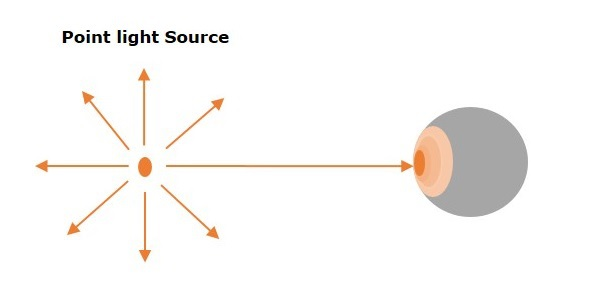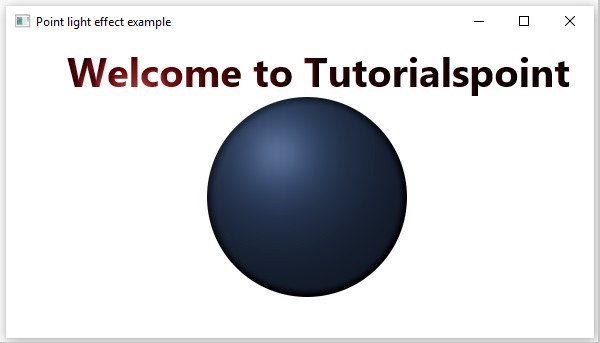# Lighting Effect - (Point Light as Source)

On applying this effect to a node, a light is simulated on it, as if it is being generated by a point light source.Point Light Source − The light from this source attenuates in all directions from a single point the intensity of the light depends on the distance of the object from the source.

The class named Point.Spot of the package javafx.scene.effect represents the point light source. This class contains four properties, which include −

• x − This property is of the type double and it represents the x coordinate of the position of the light.

• y − This property is of the type double and it represents the y coordinate of the position of the light.

• z − This property is of the type double and it represents the z coordinate of the position of the light.

## Example

The following program is an example demonstrating the lighting effect of JavaFX. In here, we are drawing a text “Welcome to Tutorialspoint” and a circle in a scene.

To these, we are applying the lighting effect, where the light is being emitted by a Point light source.

Save this code in a file with the name PointLightExample.java.

```import javafx.application.Application;
import javafx.scene.Group;
import javafx.scene.Scene;
import javafx.scene.effect.Light;
import javafx.scene.effect.Lighting;
import javafx.scene.paint.Color;
import javafx.scene.shape.Circle;
import javafx.stage.Stage;
import javafx.scene.text.Font;
import javafx.scene.text.FontWeight;
import javafx.scene.text.Text;

public class PointLightExample extends Application {
@Override
public void start(Stage stage) {
//Creating a Text object
Text text = new Text();

//Setting font to the text
text.setFont(Font.font(null, FontWeight.BOLD, 40));

//setting the position of the text
text.setX(60);
text.setY(50);

//Setting the text to be embedded.
text.setText("Welcome to Tutorialspoint");

//Setting the color of the text
text.setFill(Color.RED);

//Drawing a Circle
Circle circle = new Circle();

//Setting the center of the circle
circle.setCenterX(300.0f);
circle.setCenterY(160.0f);

//Setting the radius of the circle

//setting the fill color of the circle
circle.setFill(Color.CORNFLOWERBLUE);

//instantiating the Light.Point class
Light.Point light = new Light.Point();

//Setting the color of the light
light.setColor(Color.GRAY);

//Setting the position of the light
light.setX(70);
light.setY(55);
light.setZ(45);

//Instantiating the Lighting class
Lighting lighting = new Lighting();

//Setting the light
lighting.setLight(light);

//Applying the Lighting effect to the text
text.setEffect(lighting);

//Applying the Lighting effect to the circle
circle.setEffect(lighting);

//Creating a Group object
Group root = new Group(text,circle);

//Creating a scene object
Scene scene = new Scene(root, 600, 300);

//Setting title to the Stage
stage.setTitle("Point light effect example");

stage.setScene(scene);

//Displaying the contents of the stage
stage.show();
}
public static void main(String args[]){
launch(args);
}
}
```

Compile and execute the saved java file from the command prompt using the following commands.

```javac PointLightExample.java
java PointLightExample
```

On executing, the above program generates a JavaFX window as shown below.javafx_effects.htm